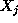Next: Feasible Regions of Two-Var Up: No Title Previous: The general LP formulation

# Graphical solution of 2-var LP's

In this section, we develop a solution approach for LP problems, which is based on a geometrical representation of the feasible region and the objective function. In particular, the space to be considered is the n-dimensional space with each dimension defined by one of the LP variables. The objective function will be described in this n-dim space by its contour plots, i.e., the sets of points that correspond to the same objective value. To the extent that the proposed approach requires the visualization of the underlying geometry, it is applicable only for LP's with upto three variables. Actually, to facilitate the visualization of the concepts involved, in this section we shall restrict ourselves to the two-dimensional case, i.e., to LP's with two decision variables. In the next section, we shall generalize the geometry introduced here for the 2-var case, to the case of LP's with n decision variables, providing more analytic (algebraic) characterizations of these concepts and properties.

UAL Data
Fri Jun 20 15:03:05 CDT 1997